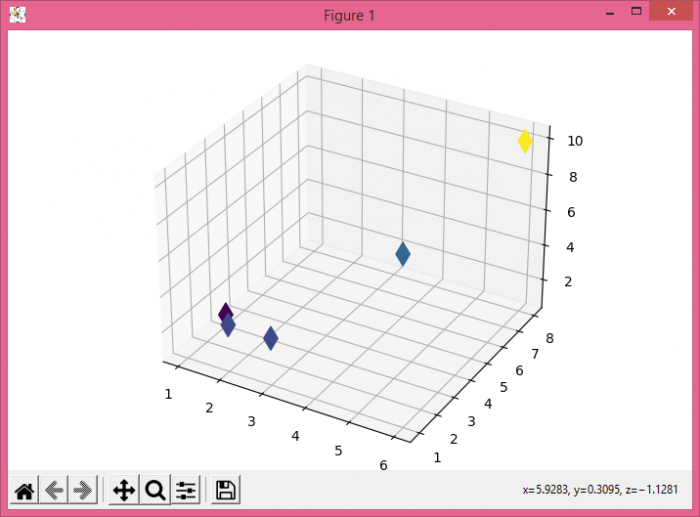# How to plot 3D graphs using Python Matplotlib?

To plot 3D graphs using Python, we can take the following steps −

• Create a new figure or activate an existing figure using figure() method.

• Get the 3D axes object.

• Make x, y, and z lists for data points.

• Add 3D scatter points using scatter3D() method, with x, y, and z data points with markersize=150 and marker=diamond.

• To display the figure, use show() method.

## Example

from mpl_toolkits.mplot3d import Axes3D
from matplotlib import pyplot as plt
plt.rcParams["figure.figsize"] = [7.00, 3.50]
plt.rcParams["figure.autolayout"] = True
fig = plt.figure()
ax = Axes3D(fig)
x = [2, 4, 6, 3, 1]
y = [1, 6, 8, 1, 3]
z = [3, 4, 10, 3, 1]
ax.scatter3D(x, y, z, c=z, alpha=1, marker='d', s=150)
plt.show()

## Output